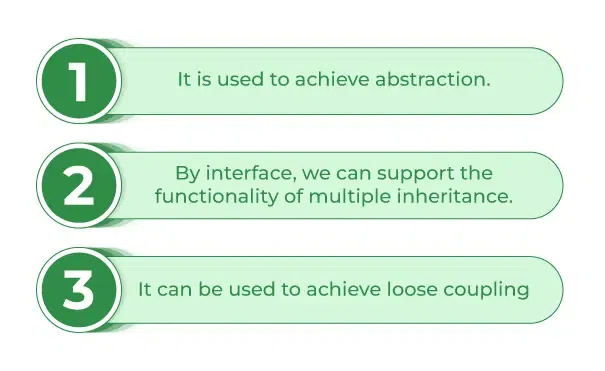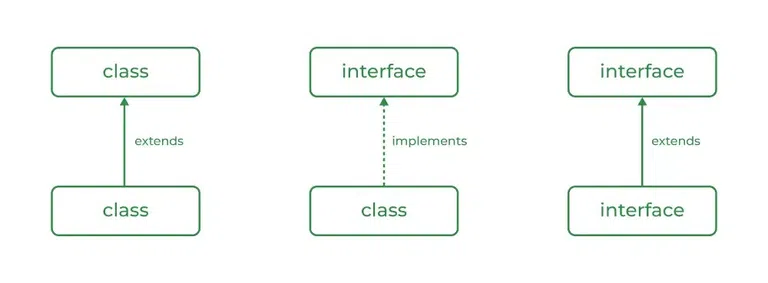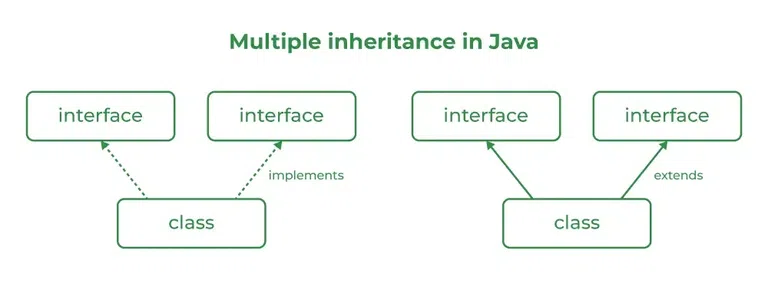# Interfaces in Java

An Interface in Java programming language is defined as an abstract type used to specify the behavior of a class. An interface in Java is a blueprint of a behavior. A Java interface contains static constants and abstract methods.

## What are Interfaces in Java?

The interface in Java is a mechanism to achieve abstraction. There can be only abstract methods in the Java interface, not the method body. It is used to achieve abstraction and multiple inheritances in Java using Interface. In other words, you can say that interfaces can have abstract methods and variables. It cannot have a method body. Java Interface also represents the IS-A relationship.

When we decide on a type of entity by its behavior and not via attribute we should define it as an interface.### Syntax for Java Interfaces

`interface {    // declare constant fields    // declare methods that abstract     // by default.   }`

To declare an interface, use the interface keyword. It is used to provide total abstraction. That means all the methods in an interface are declared with an empty body and are public and all fields are public, static, and final by default. A class that implements an interface must implement all the methods declared in the interface. To implement the interface use the implements keyword.

### Uses of Interfaces in Java

Uses of Interfaces in Java are mentioned below:

• It is used to achieve total abstraction.
• Since java does not support multiple inheritances in the case of class, by using an interface it can achieve multiple inheritances.
• Any class can extend only 1 class but can any class implement an infinite number of interface.
• It is also used to achieve loose coupling.
• Interfaces are used to implement abstraction.

So the question arises why use interfaces when we have abstract classes?

The reason is, abstract classes may contain non-final variables, whereas variables in the interface are final, public, and static.

`// A simple interfaceinterface Player{    final int id = 10;    int move();}`

## Relationship Between Class and Interface

A class can extend another class similar to this an interface can extend another interface. But only a class can extend to another interface, and vice-versa is not allowed.## Difference Between Class and Interface

Although Class and Interface seem the same there have certain differences between Classes and Interface. The major differences between a class and an interface are mentioned below:

Class

Interface

In class, you can instantiate variables and create an object. In an interface, you can’t instantiate variables and create an object.
A class can contain concrete(with implementation) methods The interface cannot contain concrete(with implementation) methods
The access specifiers used with classes are private, protected, and public. In Interface only one specifier is used- Public.

Implementation: To implement an interface we use the keyword implements

## Java

 `// Java program to demonstrate working of` `// interface`   `import` `java.io.*;`   `// A simple interface` `interface` `In1 {` `  `  `    ``// public, static and final` `    ``final` `int` `a = ``10``;`   `    ``// public and abstract` `    ``void` `display();` `}`   `// A class that implements the interface.` `class` `TestClass ``implements` `In1 {` `  `  `    ``// Implementing the capabilities of` `    ``// interface.` `    ``public` `void` `display(){ ` `      ``System.out.println(``"Geek"``); ` `    ``}`   `    ``// Driver Code` `    ``public` `static` `void` `main(String[] args)` `    ``{` `        ``TestClass t = ``new` `TestClass();` `        ``t.display();` `        ``System.out.println(a);` `    ``}` `}`

Output

```Geek
10
```

## Java Interfaces Examples

Let’s consider the example of vehicles like bicycles, cars, bikes, etc they have common functionalities. So we make an interface and put all these common functionalities. And lets Bicycle, Bike, car, etc implement all these functionalities in their own class in their own way.

Below is the implementation of the above topic:

## Java

 `// Java program to demonstrate the ` `// real-world example of Interfaces`   `import` `java.io.*;`   `interface` `Vehicle {` `    `  `    ``// all are the abstract methods.` `    ``void` `changeGear(``int` `a);` `    ``void` `speedUp(``int` `a);` `    ``void` `applyBrakes(``int` `a);` `}`   `class` `Bicycle ``implements` `Vehicle{` `    `  `    ``int` `speed;` `    ``int` `gear;` `    `  `    ``// to change gear` `    ``@Override` `    ``public` `void` `changeGear(``int` `newGear){` `        `  `        ``gear = newGear;` `    ``}` `    `  `    ``// to increase speed` `    ``@Override` `    ``public` `void` `speedUp(``int` `increment){` `        `  `        ``speed = speed + increment;` `    ``}` `    `  `    ``// to decrease speed` `    ``@Override` `    ``public` `void` `applyBrakes(``int` `decrement){` `        `  `        ``speed = speed - decrement;` `    ``}` `    `  `    ``public` `void` `printStates() {` `        ``System.out.println(``"speed: "` `+ speed` `            ``+ ``" gear: "` `+ gear);` `    ``}` `}`   `class` `Bike ``implements` `Vehicle {` `    `  `    ``int` `speed;` `    ``int` `gear;` `    `  `    ``// to change gear` `    ``@Override` `    ``public` `void` `changeGear(``int` `newGear){` `        `  `        ``gear = newGear;` `    ``}` `    `  `    ``// to increase speed` `    ``@Override` `    ``public` `void` `speedUp(``int` `increment){` `        `  `        ``speed = speed + increment;` `    ``}` `    `  `    ``// to decrease speed` `    ``@Override` `    ``public` `void` `applyBrakes(``int` `decrement){` `        `  `        ``speed = speed - decrement;` `    ``}` `    `  `    ``public` `void` `printStates() {` `        ``System.out.println(``"speed: "` `+ speed` `            ``+ ``" gear: "` `+ gear);` `    ``}` `    `  `}` `class` `GFG {` `    `  `    ``public` `static` `void` `main (String[] args) {` `    `  `        ``// creating an instance of Bicycle` `        ``// doing some operations` `        ``Bicycle bicycle = ``new` `Bicycle();` `        ``bicycle.changeGear(``2``);` `        ``bicycle.speedUp(``3``);` `        ``bicycle.applyBrakes(``1``);` `        `  `        ``System.out.println(``"Bicycle present state :"``);` `        ``bicycle.printStates();` `        `  `        ``// creating instance of the bike.` `        ``Bike bike = ``new` `Bike();` `        ``bike.changeGear(``1``);` `        ``bike.speedUp(``4``);` `        ``bike.applyBrakes(``3``);` `        `  `        ``System.out.println(``"Bike present state :"``);` `        ``bike.printStates();` `    ``}` `}`

Output

```Bicycle present state :
speed: 2 gear: 2
Bike present state :
speed: 1 gear: 1
```

## Advantages of Interfaces in Java

The advantages of using interfaces in Java are as follows:

1. Without bothering about the implementation part, we can achieve the security of the implementation.
2. In Java, multiple inheritances are not allowed, however, you can use an interface to make use of it as you can implement more than one interface.

## Multiple Inheritance in Java Using Interface

Multiple Inheritance is an OOPs concept that can’t be implemented in Java using classes. But we can use multiple inheritances in Java using Interface. let us check this with an example.Example:

## Java

 `// Java program to demonstrate How Diamond Problem` `// Is Handled in case of Default Methods`   `// Interface 1` `interface` `API {` `    ``// Default method` `    ``default` `void` `show()` `    ``{`   `        ``// Print statement` `        ``System.out.println(``"Default API"``);` `    ``}` `}`   `// Interface 2` `// Extending the above interface` `interface` `Interface1 ``extends` `API {` `}`   `// Interface 3` `// Extending the above interface` `interface` `Interface2 ``extends` `API {` `}`   `// Main class` `// Implementation class code` `class` `TestClass ``implements` `Interface1, Interface2 {` `    ``// Main driver method` `    ``public` `static` `void` `main(String args[])` `    ``{` `        ``// Creating object of this class` `        ``// in main() method` `        ``TestClass d = ``new` `TestClass();`   `        ``// Now calling the function defined in interface 1` `        ``// from whom Interface 2and 3 are deriving` `        ``d.show();` `    ``}` `}`

Output

```Default API
```

## New Features Added in Interfaces in JDK 8

There are certain features added to Interfaces in JDK 8 update mentioned below:

1. Prior to JDK 8, the interface could not define the implementation. We can now add default implementation for interface methods. This default implementation has a special use and does not affect the intention behind interfaces.

Suppose we need to add a new function to an existing interface. Obviously, the old code will not work as the classes have not implemented those new functions. So with the help of default implementation, we will give a default body for the newly added functions. Then the old codes will still work.

Below is the implementation of the above point:

## Java

 `// Java program to show that interfaces can` `// have methods from JDK 1.8 onwards`   `interface` `In1` `{` `    ``final` `int` `a = ``10``;` `    ``default` `void` `display()` `    ``{` `        ``System.out.println(``"hello"``);` `    ``}` `}`   `// A class that implements the interface.` `class` `TestClass ``implements` `In1` `{` `    ``// Driver Code` `    ``public` `static` `void` `main (String[] args)` `    ``{` `        ``TestClass t = ``new` `TestClass();` `        ``t.display();` `    ``}` `}`

Output

```hello
```

2. Another feature that was added in JDK 8 is that we can now define static methods in interfaces that can be called independently without an object.

Note: these methods are not inherited.

## Java

 `// Java Program to show that interfaces can` `// have methods from JDK 1.8 onwards`   `interface` `In1` `{` `    ``final` `int` `a = ``10``;` `    ``static` `void` `display()` `    ``{` `        ``System.out.println(``"hello"``);` `    ``}` `}`   `// A class that implements the interface.` `class` `TestClass ``implements` `In1` `{` `    ``// Driver Code` `    ``public` `static` `void` `main (String[] args)` `    ``{` `        ``In1.display();` `    ``}` `}`

Output

```hello
```

## Extending Interfaces

One interface can inherit another by the use of keyword extends. When a class implements an interface that inherits another interface, it must provide an implementation for all methods required by the interface inheritance chain.

Program 1:

## Java

 `interface` `A {` `    ``void` `method1();` `    ``void` `method2();` `}` `// B now includes method1 and method2` `interface` `B ``extends` `A {` `    ``void` `method3();` `}` `// the class must implement all method of A and B.` `class` `gfg ``implements` `B {` `    ``public` `void` `method1()` `    ``{` `        ``System.out.println(``"Method 1"``);` `    ``}` `    ``public` `void` `method2()` `    ``{` `        ``System.out.println(``"Method 2"``);` `    ``}` `    ``public` `void` `method3()` `    ``{` `        ``System.out.println(``"Method 3"``);` `    ``}` `}`

Program 2:

## Java

 `interface` `Student  ` `{` `    ``public` `void` `data();` `   `  `}` `class` `avi ``implements` `Student` `{` `    ``public` `void` `data ()` `    ``{` `        ``String name=``"avinash"``;` `        ``int` `rollno=``68``;` `        ``System.out.println(name);` `        ``System.out.println(rollno);` `    ``}` `}` `public` `class` `inter_face ` `{` `    ``public` `static` `void` `main (String args [])` `    ``{` `        ``avi h= ``new` `avi();` `        ``h.data();` `    ``}` `}`

Output

```avinash
68
```

In a Simple way, the interface contains multiple abstract methods, so write the implementation in implementation classes. If the implementation is unable to provide an implementation of all abstract methods, then declare the implementation class with an abstract modifier, and complete the remaining method implementation in the next created child classes. It is possible to declare multiple child classes but at final we have completed the implementation of all abstract methods.

In general, the development process is step by step:

1. Level 1-  interfaces: It contains the service details.

2. Level 2 – abstract classes: It contains partial implementation.

3. Level 3 – implementation classes: It contains all implementation.

4. Level 4 – Final Code / Main Method: It have access of all interfaces data.

Example:

## Java

 `// Java Program for` `// implementation Level wise` `import` `java.io.*;` `import` `java.lang.*;` `import` `java.util.*;`   `// Level 1` `interface` `Bank {` `    ``void` `deposit();` `    ``void` `withdraw();` `    ``void` `loan();` `    ``void` `account();` `}`   `// Level 2` `abstract` `class` `Dev1 ``implements` `Bank {` `    ``public` `void` `deposit()` `    ``{` `        ``System.out.println(``"Your deposit Amount :"` `+ ``100``);` `    ``}` `}`   `abstract` `class` `Dev2 ``extends` `Dev1 {` `    ``public` `void` `withdraw()` `    ``{` `        ``System.out.println(``"Your withdraw Amount :"` `+ ``50``);` `    ``}` `}`   `// Level 3` `class` `Dev3 ``extends` `Dev2 {` `    ``public` `void` `loan() {}` `    ``public` `void` `account() {}` `}`   `// Level 4` `class` `GFG {` `    ``public` `static` `void` `main(String[] args)` `    ``{` `        ``Dev3 d = ``new` `Dev3();` `        ``d.account();` `        ``d.loan();` `        ``d.deposit();` `        ``d.withdraw();` `    ``}` `}`

Output

```Your deposit Amount :100
```

## New Features Added in Interfaces in JDK 9

From Java 9 onwards, interfaces can contain the following also:

1. Static methods
2. Private methods
3. Private Static methods

## Important Points in Java Interfaces

In the article, we learn certain important points about interfaces as mentioned below:

• We can’t create an instance(interface can’t be instantiated) of the interface but we can make the reference of it that refers to the Object of its implementing class.
• A class can implement more than one interface.
• An interface can extend to another interface or interface (more than one interface).
• A class that implements the interface must implement all the methods in the interface.
• All the methods are public and abstract. And all the fields are public, static, and final.
• It is used to achieve multiple inheritances.
• It is used to achieve loose coupling.
• Inside the Interface not possible to declare instance variables because by default variables are public static final.
• Inside the Interface, constructors are not allowed.
• Inside the interface main method is not allowed.
• Inside the interface, static, final, and private methods declaration are not possible.

## Frequently Asked Questions in Interfaces

### 1. What is a marker or tagged interface?

Tagged Interfaces are interfaces without any methods they serve as a marker without any capabilities.

### 2. How many Types of interface in Java?

Types of interfaces in Java are mentioned below:

1. Functional Interface
2. Marker interface

### 3.  Why multiple inheritance is not supported through class in Java?

Multiple Inheritance is not supported through class in Java so to avoid certain challenges like Ambiguity and diamond problems.